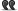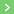règle de simplification

# règle de simplification

1. ## règle de simplification

------

Hi,

Do you know the translation of: "règle de simplification"?

"simplification rule"

or

"reduction rule"

Best. Th.

-----2. ## Re : règle de simplification

For example:

"Calculation rules" and properties of the addition, multiplication and order in the (commutative) semiring of natural numbers are presented in following tables:

Associativity: (n+m)+k=n+(m+k)
Commutativity: n+m=m+n
Neutral element: n+0=0+n=n
Monotony: n≤m is equivalent to n+k≤m+k
Reduction rule: n+k=m+k implies n=m
Distributivity: k(m+n)=km+kn

Multiplication
Associativity: (nm)k=n(mk)
Commutativity: nm=mn
Neutral element: n.1=1.n=n
Monotony: k>0 implies (n≤m equivalents to nk≤mk)
Reduction rule: n≠0 implies (nk=mk implies n=m)
Distributivity: k(m+n)=km+kn

reduction or simplification ? thanks3. ## Re : règle de simplificationEnvoyé par th..For example:

"Calculation rules" and properties of the addition, multiplication and order in the (commutative) semiring of natural numbers are presented in following tables:

Associativity: (n+m)+k=n+(m+k)
Commutativity: n+m=m+n
Neutral element: n+0=0+n=n
Monotony: n≤m is equivalent to n+k≤m+k
Reduction rule: n+k=m+k implies n=m
Distributivity: k(m+n)=km+kn

Multiplication
Associativity: (nm)k=n(mk)
Commutativity: nm=mn
Neutral element: n.1=1.n=n
Monotony: k>0 implies (n≤m equivalents to nk≤mk)
Simplification rule: k≠0 implies (nk=mk implies n=m)
Distributivity: k(m+n)=km+kn

reduction or simplification ? thanks
Isn't it ?
I'm certain that "règle de simplification" means "simplification rule", maybe it means "reduction rule" too ^o)4. ## Re : règle de simplificationEnvoyé par kNzIsn't it ?
I'm certain that "règle de simplification" means "simplification rule", maybe it means "reduction rule" too ^o)
Are you sure? Or is it a joke?

(ps: where is Jimbaran?)